### Home > CC1MN > Chapter 3 > Lesson 3.2.2 > Problem3-108

3-108.

In parts (a) and (b) below, copy the vertical number lines and use them to show the solution to the problems.

1. $5 − 2$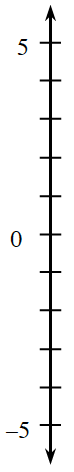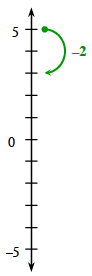$3$

1. $2 + 1$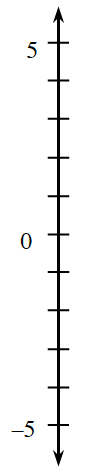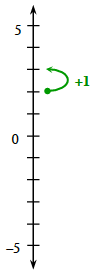1. Show how to find the answer to $−3 + 4 − 1$ on a number line.

• See parts (a) and (b) for help.

1. Rachel said that $−4 −3$ ends up being positive because two negatives always give a positive. Draw the problem on a number line, and then explain whether you agree or disagree with Rachel.

•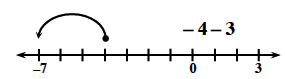$−1 + (−2) = −3$
$−7 + (−4) = −11$
Do two negatives always make a positive?